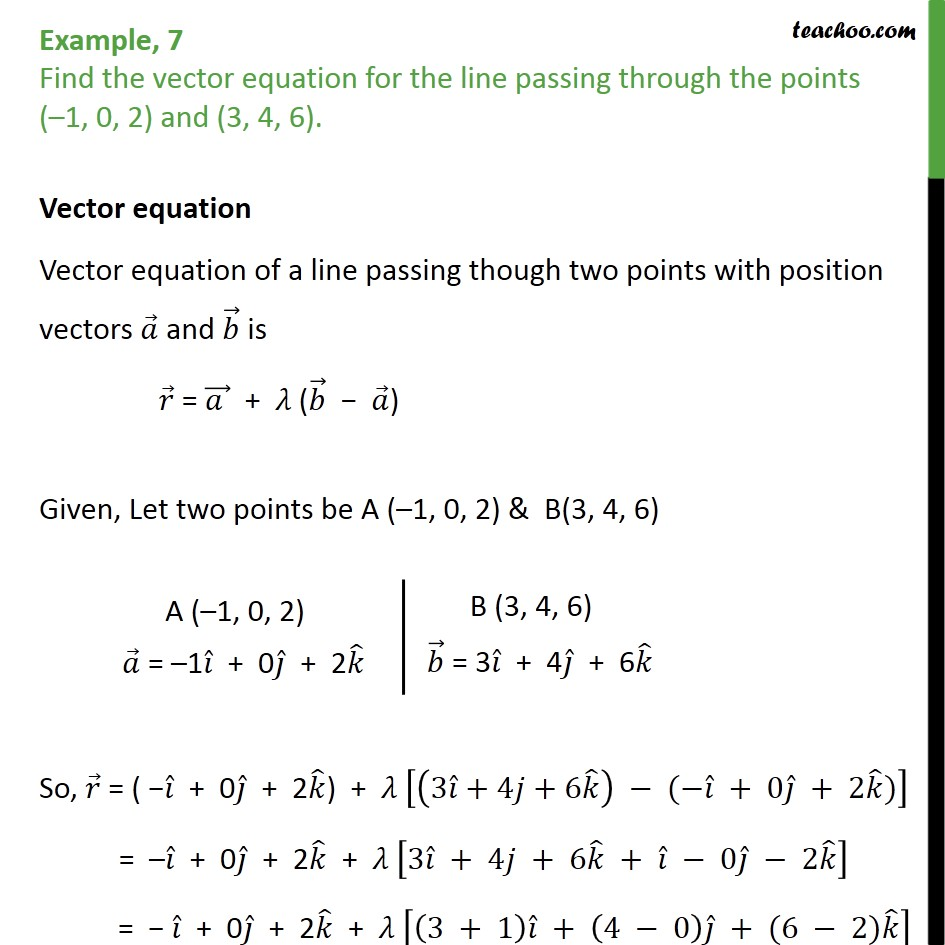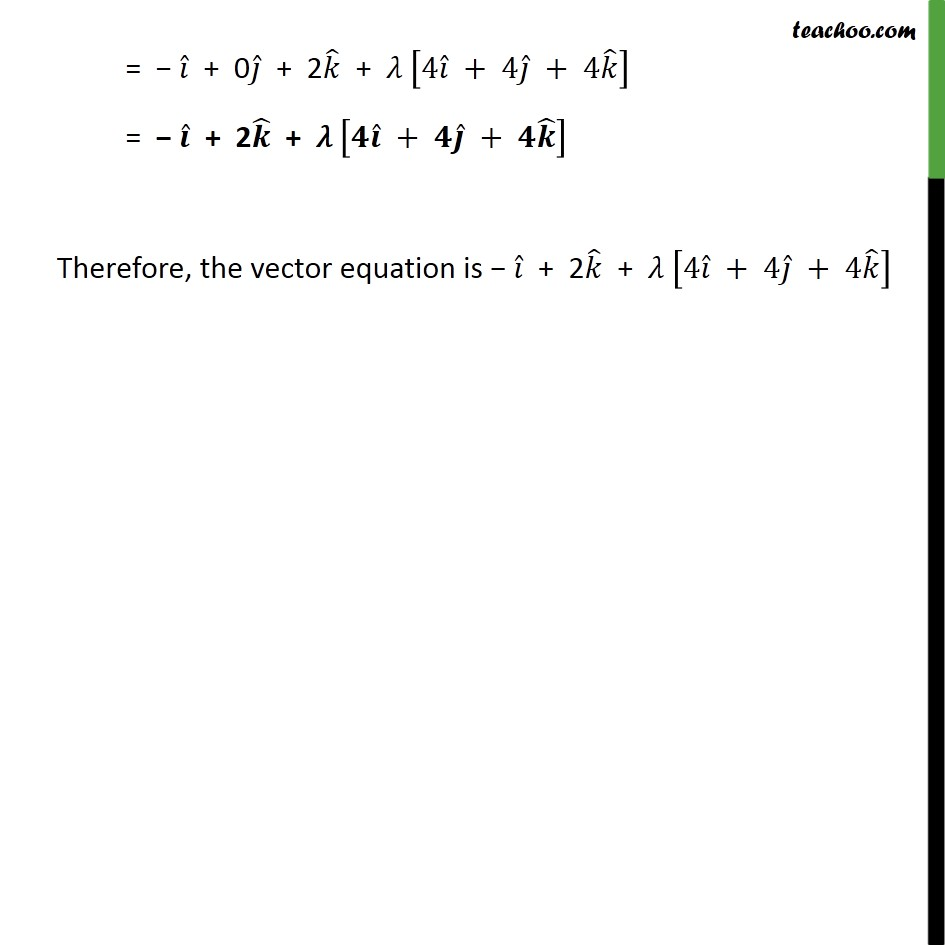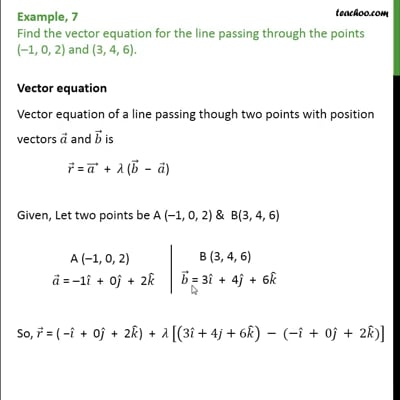Examples

Chapter 11 Class 12 Three Dimensional Geometry
Serial order wiseThis video is only available for Teachoo black users

Get live Maths 1-on-1 Classs - Class 6 to 12

### Transcript

Example, 7 Find the vector equation for the line passing through the points ( 1, 0, 2) and (3, 4, 6). Vector equation Vector equation of a line passing though two points with position vectors and is = + ( ) Given, Let two points be A ( 1, 0, 2) & B(3, 4, 6) So, = ( + 0 + 2 ) + 3 +4 +6 ( + 0 + 2 ) = + 0 + 2 + 3 + 4 + 6 + 0 2 = + 0 + 2 + 3 + 1 + 4 0 + (6 2) = + 0 + 2 + 4 + 4 + 4 = + 2 + + + Therefore, the vector equation is + 2 + 4 + 4 + 4